# 4 steps to get the risk free interest rates term structures provided by EIOPA di Silvia Dell’Acqua

Apr 16 2015Under Solvency II the whole economic balance sheet has to be evaluated according to market consistent principles and to this end, EIOPA publishes on a monthly basis the relevant risk free interest rates term structures. Following the Omnibus 2 directive (2014/51/EU), EIOPA has also published a Technical Document with all the assumptions and methodologies adopted provided in a transparent manner: in general a low degree of expert judgment and data from deep and transparent financial markets are used to let insurance and reinsurance undertakings replicate the calculus.

The risk free rates curves can be derived following these 4 steps:

1. Consider appropriate inputs and providers

–       Markit – iBoxx indices to download bonds other than government or other assets

The basic risk free rates interest rates term structures are defined from maturity 1y onward and are derived by swap rates or, if not available or not sufficiently reliable, by government bond rates of the country; mid prices are adopted. Two examples of the financial instrument considered are EUSA CMPN Curncy – EUR006M Index for the Euro currency, and BPSW CMPN Curncy – BP0006M Index for the GBP one.

2. Apply the Credit Risk Adjustment (CRA) as a parallel downward (to observed par swap rates or observed government zero coupon rates) up to the Last Liquid Point (LLP) to reflect the risk of default of the counterparty. The LPP is considered to be the longest maturity that meets the Deep, Liquid and Transparent (DLT) requirements; the assessment has been performed by all the National Competent Authorities for the Euro currency, by each member state for the other EEA currencies and by EIOPA itself for the non-EEA currencies. For the Euro, the LLP is set to meet the residual volume criteria: the cumulative volume of bonds with maturities ≥ the LLP is less than 6% of the volume of all the bonds in the market. The LLP is set to 20y for the Euro and currencies pegged to the Euro; it is 50y for GBP.

CRA is calculated as the 50% of the average over 1y of the differences between the floating rate of interest rate swaps and the Overnight Index Swap (OIS) rates of the same maturity; it has to belong to the range [10-35] bps. For the Euro the OIS to be considered is the 3m rate (EUR003M Index – EUSWEC CMPN Curncy) while for the United Kingdom it is the 6m one (BP0006M Index – BPSWSF CMPN Curncy).

For currencies pegged to the Euro (CPEA) apply a currency adjustment as a second downward shift. Both these adjustments may lead to negative interest rates.

3. Extrapolate (and also interpolate if necessary) interest rates from the last point at which data are available (LLP) to a macroeconomic long term equilibrium rate (Ultimate Forward Rate – UFR) applying the Smith Wilson technique.

The alpha parameter that controls the convergence speed is set as the lowest value which makes the curve reach the UFW with a tolerance of 1bps. A lower bound for alpha is set to 0.05.

The UFR corresponds to a convergence maturity of 60y for the Euro and of max(60y,LLP+40y) for other currencies; it is in general equal to 4.20%, but for CHF and JPY (3.20%) and for Brazilian, Indian, Mexican, Turkish and South Africans currencies (5.20%).

4.Apply the Volatility Adjustment (VA) till the LLP to reduce the impact of short term market volatility on the balance sheet of undertakings; the influence at group level must be derived from the VA adjustment applied at each component of the group. The VA expresses a portion (65%) of the spread between the interest rates that could be earned from assets included in a reference portfolio and the basic risk free rates. It is defined at currency level and might be corrected at country level if the country increase in the volatility is significant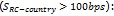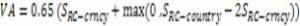where

–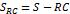is the risk corrected spread (that may be negative) calculated as the difference between the spread  and the risk correction , the latter is also known as Fundamental Spread (FS)

–    s   is the spread between the interest rates derived from the reference portfolio and the basic interest rate term structure. It is calculated as a weighted average of the average spreads of governments and corporate bonds included in the reference portfolio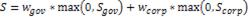–    RC  is the portion of the spread actually at risk (realistic assessment of expected losses, unexpected credit risk or any other risk) in the reference portfolio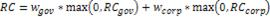–       examples of weights for the preparatory phase in 2015 are: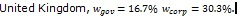For each relevant currency (or country) the spread before the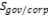and the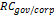are derived as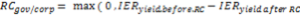where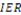is for Internal Effective Rate of the overall reference portfolio, calculated as the single discount rate that applied to the cash flows of the whole portfolio equals its aggregate value. Each single cash flow of the portfolio is projected according to the duration of the model bond with the capitalization rate explicated above (basic risk free rate / yield before the RC / yield after the RC). In the case of Euro currency, all the government curves of the members of the Euro zone are replaced by a single curve, the ECB one.

For each model bond of the reference portfolio the Risk Correction is calculated as follows, in the same manner as for the Matching Adjustment (MA) Fundamental Spread – see below:

–       the RC linked to sovereign debt is a percentage of the Long Term Average Spread (LTAS) over the basic risk free curves (30% LTAS for exposures to Member States’ central governments and central banks, 35% for non Member); the LTAS should be based on data referring to the last 30y, but, being these not available, the following formula is adopted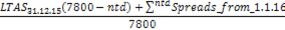7800 are the trading days in a 30y period and  denotes the number of new trading days from 1.1.2016 and. To avoid any bias, LATS for government bonds is carried out from 1 to 10y maturities and then applied as a constant for longer maturities. Examples of financial instruments used for the derivation of the VA and MA regarding sovereign bonds are: Italy, I040 CMPN Index; Germany, I016 CMPN Index; United Kingdom, I022 CMPN Index.

–       For other assets the RC is based on the maximum between a percentage of the LTAS and the sum  of the credit spread corresponding to a Probability of Default (PD) and the Costs of Downgrade (CoD) based on the projection of an average 1y transition matrix. 2y LTAS is used for 1y LTAS also and for the last maturity available onward the last LTAS is used as a constant. In case of missing LTAS, interpolation is used among the available maturities. The calculation of the PD derives an amount that is interpreted as the compensation required by the investor for assuming the risk of expected default of the bond , assuming a Recovery Rate RR=30% of the Market Value. While the PD spread computation does not consider the cost accounting, the CoD one uses adjusted transition matrix. The CoD reflects the cost of replacing the downgraded asset with an asset of the same credit quality as the initial one and is applied until the maturity of the bond: the total loss is transformed into an implied higher yield and then expressed as a spread (in bps) over the basic risk free interest rate. The scaling factors to be considered for each credit quality step are

 Credit Quality Step Scaling factor Credit Quality Step Scaling factor AAA 98% BB 70% AA 97% BB 50% A 95% CCC 40% BBB 85%

Only two transition matrices are considered (financial and not financial), with the 1y transition average calculated over the last 30 years until 1.1.2014.

Further notes on the VA:

–       it is rounded to the nearest integer basis point and applied to all maturities till the LLP

–       it is not added to the par swap rates adjusted for the CRA, but it is added to the zero coupon spot rates of the basic risk free interest rate term structure obtained after using the Smith Wilson method

–       the resulting curve is then extrapolated using the Smith Wilson technique and, therefore, the relevant risk free rate term structure included the VA is a parallel shift over the basic one only until the LLP; after this maturity both converge to the same UFR.

The Matching Adjustment is made in respect of a predictable portfolios of liabilities whose assets are characterized by fixed cash flows and are held to maturity; it is applied as a parallel shift on the whole basic risk free curve to decrease the spread of the assets given the lower risk will faced since they are held till maturity. EIOPA publishes only the fundamental spread to be used and then undertakings must calculate the MA themselves.

I commenti per questo post sono chiusi